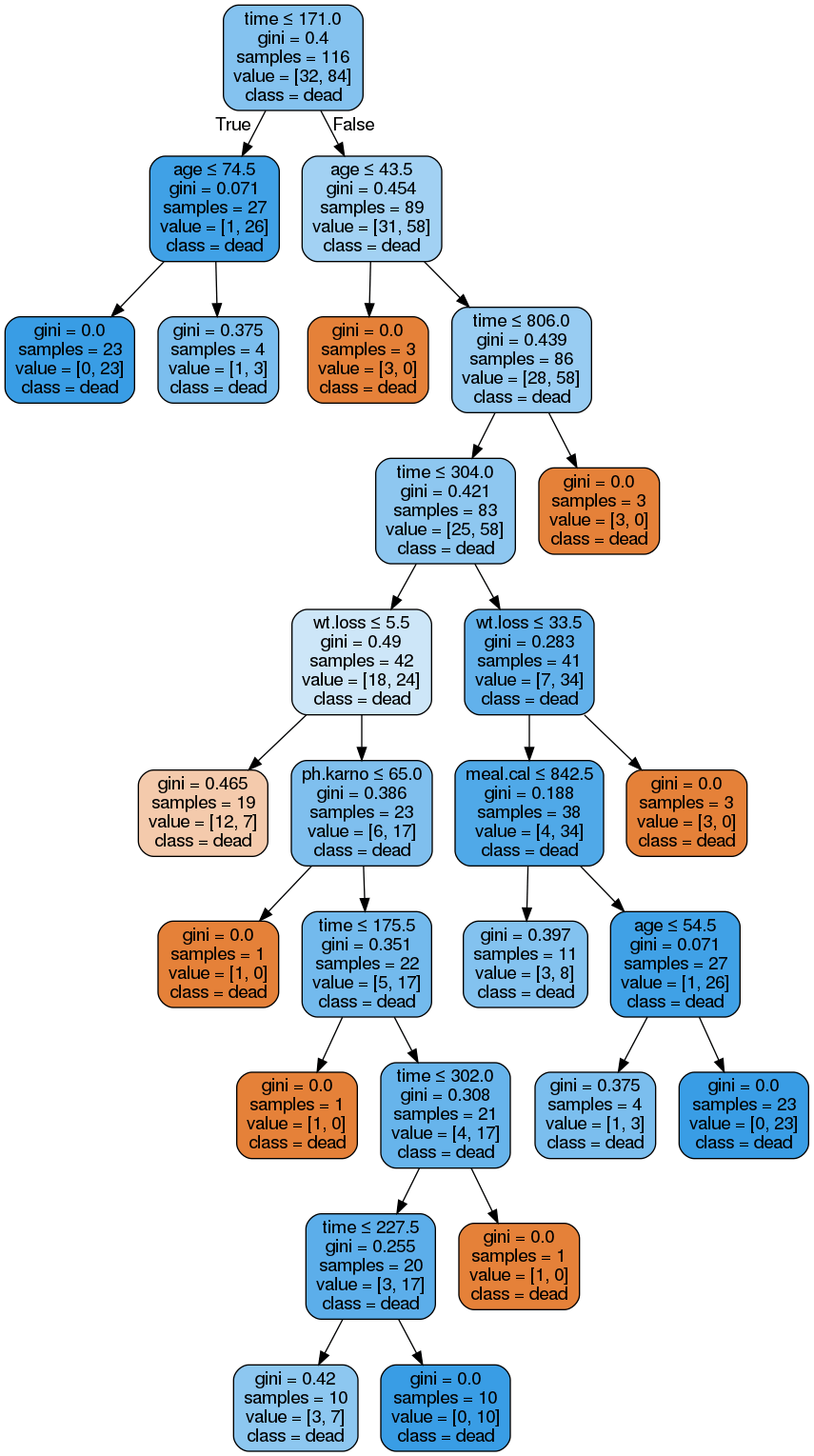# Decision Trees in Python

Decision trees are used in machine learning. They are easy to understand and are able to deal with data that is less than ideal. In addition, because of the pictorial nature of the results decision trees are easy for people to interpret.We are going to use the ‘cancer’ dataset to predict mortality based on several independent variables.

We will follow the steps below for our decision tree analysis

1. Data preparation
2. Model development
3. Model evaluation

Data Preparation

We need to load the following modules in order to complete this analysis.

```import pandas as pd
import statsmodels.api as sm
import sklearn
from pydataset import data
from sklearn.model_selection import train_test_split
from sklearn import metrics
from sklearn import tree
import matplotlib.pyplot as plt
from sklearn.externals.six import StringIO
from IPython.display import Image
from sklearn.tree import export_graphviz
import pydotplus```

The ‘cancer’ dataset comes from the ‘pydataset’ module. You can learn more about the dataset by typing the following

`data('cancer', show_doc=True)`

This provides all you need to know about our dataset in terms of what each variable is measuring. We need to load our data as ‘df’. In addition, we need to remove rows with missing values and this is done below.

```df = data('cancer')
len(df)
Out: 228
df=df.dropna()
len(df)
Out: 167```

The initial number of rows in the data set was 228. After removing missing data it dropped to 167. We now need to setup up our lists with the independent variables and a second list with the dependent variable. While doing this, we need to recode our dependent variable “status” so that the numerical values are replaced with a string. This will help us to interpret our decision tree later. Below is the code

```X=df[['time','age',"sex","ph.ecog",'ph.karno','pat.karno','meal.cal','wt.loss']]
df['status']=df.status.replace(1,'censored')
y=df['status']```

Next,  we need to make our train and test sets using the train_test_split function.  We want a 70/30 split. The code is below.

`x_train, x_test, y_train, y_test = train_test_split(X, y, test_size=0.3, random_state=0)`

We are now ready to develop our model.

Model Development

The code for the model is below

```clf=tree.DecisionTreeClassifier(min_samples_split=10)
clf=clf.fit(x_train,y_train)```

We first make an object called “clf” which calls the DecisionTreeClassifier. Inside the parentheses, we tell Python that we do not want any split in the tree to contain less than 10 examples. The second “clf” object uses the  .fit function and calls the training datasets.

We can also make a visual of our decision tree.

```dot_data = StringIO()
export_graphviz(clf, out_file=dot_data,
filled=True, rounded=True,feature_names=list(x_train.columns.values),
special_characters=True)
graph = pydotplus.graph_from_dot_data(dot_data.getvalue())
Image(graph.create_png())```If we interpret the nodes furthest to the left we get the following

• If a person has had cancer less than 171 days and
• If the person is less than 74.5 years old then

If you look closely every node is classified as ‘dead’ this may indicate a problem with our model. The evaluation metrics are below.

Model Evaluation

We will use the .crosstab function and the metrics classification functionsYou can see that the metrics are not that great in general. This may be why everything was classified as ‘dead’. Another reason is that few people were classified as ‘censored’ in the dataset.

Conclusion

Decisions trees are another machine learning tool. Python allows you to develop trees rather quickly that can provide insights into how to take action.